# sklearn.naive_bayes.GaussianNB¶

class sklearn.naive_bayes.GaussianNB(priors=None, var_smoothing=1e-09)[source]

Gaussian Naive Bayes (GaussianNB)

Can perform online updates to model parameters via partial_fit method. For details on algorithm used to update feature means and variance online, see Stanford CS tech report STAN-CS-79-773 by Chan, Golub, and LeVeque:

Read more in the User Guide.

Parameters: priors : array-like, shape (n_classes,) Prior probabilities of the classes. If specified the priors are not adjusted according to the data. var_smoothing : float, optional (default=1e-9) Portion of the largest variance of all features that is added to variances for calculation stability. class_prior_ : array, shape (n_classes,) probability of each class. class_count_ : array, shape (n_classes,) number of training samples observed in each class. theta_ : array, shape (n_classes, n_features) mean of each feature per class sigma_ : array, shape (n_classes, n_features) variance of each feature per class epsilon_ : float absolute additive value to variances

Examples

>>> import numpy as np
>>> X = np.array([[-1, -1], [-2, -1], [-3, -2], [1, 1], [2, 1], [3, 2]])
>>> Y = np.array([1, 1, 1, 2, 2, 2])
>>> from sklearn.naive_bayes import GaussianNB
>>> clf = GaussianNB()
>>> clf.fit(X, Y)
GaussianNB(priors=None, var_smoothing=1e-09)
>>> print(clf.predict([[-0.8, -1]]))

>>> clf_pf = GaussianNB()
>>> clf_pf.partial_fit(X, Y, np.unique(Y))
GaussianNB(priors=None, var_smoothing=1e-09)
>>> print(clf_pf.predict([[-0.8, -1]]))



Methods

 fit(self, X, y[, sample_weight]) Fit Gaussian Naive Bayes according to X, y get_params(self[, deep]) Get parameters for this estimator. partial_fit(self, X, y[, classes, sample_weight]) Incremental fit on a batch of samples. predict(self, X) Perform classification on an array of test vectors X. predict_log_proba(self, X) Return log-probability estimates for the test vector X. predict_proba(self, X) Return probability estimates for the test vector X. score(self, X, y[, sample_weight]) Returns the mean accuracy on the given test data and labels. set_params(self, \*\*params) Set the parameters of this estimator.
__init__(self, priors=None, var_smoothing=1e-09)[source]
fit(self, X, y, sample_weight=None)[source]

Fit Gaussian Naive Bayes according to X, y

Parameters: X : array-like, shape (n_samples, n_features) Training vectors, where n_samples is the number of samples and n_features is the number of features. y : array-like, shape (n_samples,) Target values. sample_weight : array-like, shape (n_samples,), optional (default=None) Weights applied to individual samples (1. for unweighted). New in version 0.17: Gaussian Naive Bayes supports fitting with sample_weight. self : object
get_params(self, deep=True)[source]

Get parameters for this estimator.

Parameters: deep : boolean, optional If True, will return the parameters for this estimator and contained subobjects that are estimators. params : mapping of string to any Parameter names mapped to their values.
partial_fit(self, X, y, classes=None, sample_weight=None)[source]

Incremental fit on a batch of samples.

This method is expected to be called several times consecutively on different chunks of a dataset so as to implement out-of-core or online learning.

This is especially useful when the whole dataset is too big to fit in memory at once.

This method has some performance and numerical stability overhead, hence it is better to call partial_fit on chunks of data that are as large as possible (as long as fitting in the memory budget) to hide the overhead.

Parameters: X : array-like, shape (n_samples, n_features) Training vectors, where n_samples is the number of samples and n_features is the number of features. y : array-like, shape (n_samples,) Target values. classes : array-like, shape (n_classes,), optional (default=None) List of all the classes that can possibly appear in the y vector. Must be provided at the first call to partial_fit, can be omitted in subsequent calls. sample_weight : array-like, shape (n_samples,), optional (default=None) Weights applied to individual samples (1. for unweighted). New in version 0.17. self : object
predict(self, X)[source]

Perform classification on an array of test vectors X.

Parameters: X : array-like, shape = [n_samples, n_features] C : array, shape = [n_samples] Predicted target values for X
predict_log_proba(self, X)[source]

Return log-probability estimates for the test vector X.

Parameters: X : array-like, shape = [n_samples, n_features] C : array-like, shape = [n_samples, n_classes] Returns the log-probability of the samples for each class in the model. The columns correspond to the classes in sorted order, as they appear in the attribute classes_.
predict_proba(self, X)[source]

Return probability estimates for the test vector X.

Parameters: X : array-like, shape = [n_samples, n_features] C : array-like, shape = [n_samples, n_classes] Returns the probability of the samples for each class in the model. The columns correspond to the classes in sorted order, as they appear in the attribute classes_.
score(self, X, y, sample_weight=None)[source]

Returns the mean accuracy on the given test data and labels.

In multi-label classification, this is the subset accuracy which is a harsh metric since you require for each sample that each label set be correctly predicted.

Parameters: X : array-like, shape = (n_samples, n_features) Test samples. y : array-like, shape = (n_samples) or (n_samples, n_outputs) True labels for X. sample_weight : array-like, shape = [n_samples], optional Sample weights. score : float Mean accuracy of self.predict(X) wrt. y.
set_params(self, **params)[source]

Set the parameters of this estimator.

The method works on simple estimators as well as on nested objects (such as pipelines). The latter have parameters of the form <component>__<parameter> so that it’s possible to update each component of a nested object.

Returns: self

## Examples using sklearn.naive_bayes.GaussianNB¶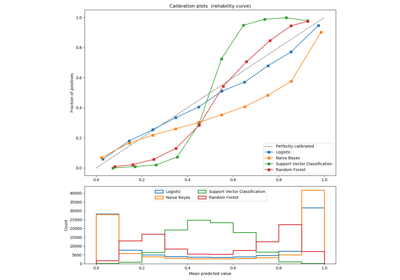Comparison of Calibration of Classifiers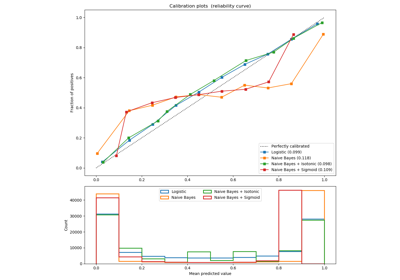Probability Calibration curves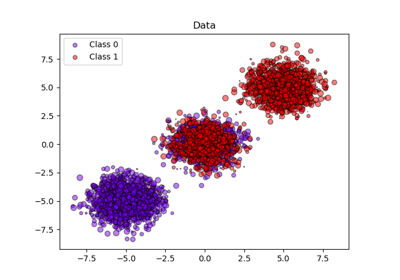Probability calibration of classifiers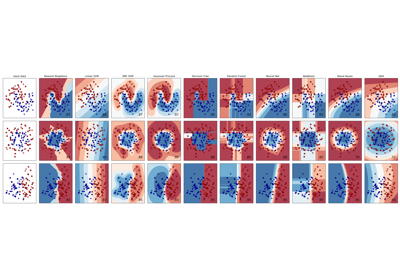Classifier comparison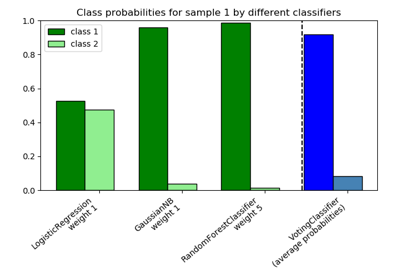Plot class probabilities calculated by the VotingClassifier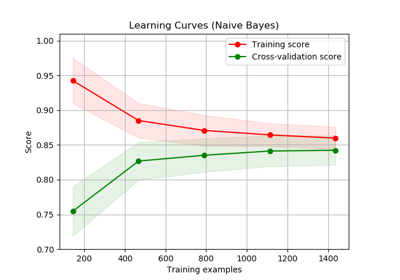Plotting Learning Curves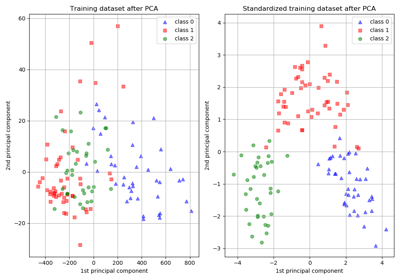Importance of Feature Scaling